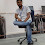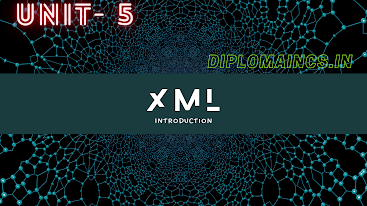## 1/9/20

### Previous year question for Computer Science and Engineering semester 4 Digital electronics and microprocessor year 2018

Sub Code: - 1618402

2018(Even)
Time : 3Hrs
Semester  IV(New)
DE & M

Full Marks : 70
Pass marks : 28

Group A
Choose the most suitable answer from the following options: -   (1*20=20)

(i) Truth table is used to express:

(a) Boolean expression
(b) Boolean map
(c) Boolean matrix

(ii) 1’s complement of 11001010 is given by:

(a) 11001011
(b) 11001001
(c) 00110101
(d) 00110111

(iii) The time required for a gate or inverter to change its state called:

(a) Rise time
(b) Decay time
(c) Propagation time
(d) Charging time

(v) A microprocessor is ALU and:
(a) Control unit on a single chip
(b) Memory on a single chip
(c) Register unit and I/O device on a single chip
(d) Register unit and control unit on a single chip

(vii) K- map is used for the purpose:
(a) Of reducing the electronic circuits used
(b) To map the given Boolean logic function
(c) To minimize the terms in a Boolean expression
(d) To maximize the terms of a given Boolean expression

(viii) EPROM contents can be erased by exposing it to:

(a) U-V rays
(b) Infra red rays
(d) None of the above

(ix) The gates required to build a half adder are:

(a) EX-OR gate and NOR gate
(b) EX-OR gate and OR gate
(c) EX-OR gate and AND gate
(d) Four NAND gate

(x)  The number of output pins of a 8085 microprocessor are:

(a) 40
(b) 27
(c) 21
(d) 19

(xi) Which one of the following is 8-bit register in microprocessor?

(a) 16-but stack pointer (SP)
(b) 16-bit program counter (SC)
(c) Accumulator
(d) None of the above

(xii) General purpose registers in a 8085 microprocessor are:

(a) B-C, D-E &H-L
(b) B-C and H-L
(c) A, B-C, H-L
(d) None of the above

(xiii) The use of OP-AMP is generally not preferred as:

(a) Divider
(b) Integrator
(c) Subtractor
(d) Differentiator

(xiv) Which of the following is a universal gate?

(a) NOT
(b) AND
(c) NOR
(d) OR

(xv) In Boolean Algebra the plus sign (+) indicates:

(a) AND Operation
(b) OR Operation
(c) NOT Operation
(d) None of the above

(xvi) The unit that performs the arithmetic and logical operation on the stored number in known as:

(a) Arithmetic logic unit
(b) Control unit
(c) Memory
(d) Processor

(xvii) LED’s are usually switched ON and OFF by:

(a) Resistor in the circuit
(b) Diode in the circuit
(c) Transistor circuit
(d) None of the above

(xviii) A stack pointer is  _______ bit resistor in 8085 microprocessor.

(a) 8 bit
(b) 12 bit
(c) 16 bit
(d) 32 bit

(xix) Flip-flops come in the category of:

(a) Combinational logic circuit
(b) Sequential logic circuit
(c) Both the combinational as well as sequential logic circuit
(d) None of the above

(xx) The solution of (10000-1111) is given by:

(a) 1001
(b) 0001
(c) 1110
(d) 0000

Group:-"B"

Answer all Five Questions: -                         (5*4=20)

2.  (a) What is the difference between binary code and BCD? Explain.
(b) Convert the decimal number 1245 into octal.
OR
Solve the following problem given in binary forms:

3.  Simplify the following and realize it using NOR gate only:

4. What is De Morgan's theorem? Prove the following using De Morgan's theorem:

OR
Draw the logic symbol and explain the operation of T-type flip-flop.

5.  What are the essential elements of a CPU? Discuss the function of each element in brief.
OR
Explain the operation of program counter in 8085 microprocessor.

6. Define combinational and sequential logical circuits. 2+2 zorS191 Also give examples of each.
OR
Write a short note on Master-Slave J-K flip-flop.

Group:- "C"

Answer all Five Questions: -                         (5*6=30)

7.  Explain Seven segment display with diagram.
OR
a) What is an excess-3 code?
(b) Add the following hex numbers
1.  93 + DE
2. ABCD + EF12

8.  What do you mean by Min-terms and Max-terms of Boolean expression?
OR
Draw the K-map for the given function:

9.  What is A/D converter? Explain; also explain any one type of A/D converter.
OR
Explain the operation of a 4-bit binary counter with necessary waveforms.

10.  Explain the operations performed by following instructions:
CMPM, RAR, CMA and POP rp
OR
What do you understand by (a) main memory and (b) secondary memory? Explain.

11.  Explain the function of following pins in the schematic diagram of Intel 8085 µP.

OR
Draw a full adder circuit and explain its operation with truth table.

(Note:- Update available soon, comment for any type of help)

Data Structure using C: -Click me
Object Oriented Programming C++: -Click me
Data base management system: - Click me
System Analysis and management information system- Click me
Digital Electronics and microprocessor: -Click me

Semester 3rd previous year question

Computer Organization and Architecture  : - Click me
Operating System:-  Click me
Introduction to Software Package: - Click me
Computer Programing Through 'C':- Click me

Semester 5th previous year question
Internet and Web Technologies - Software Engineering : - Click me
System maintenance: - Click me
Data Communication and Networking : - Click me
JAVA: - Click me

1.Tq sir
Easy to understand sir

1.You are welcome..!!

Please do not enter any spam link in the comment box and use English and Hindi language for comment.

## Latest Update

### Key Components of XML